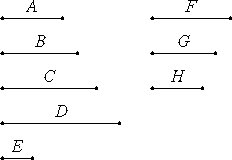Proposition 12

If as many numbers as we please beginning from a unit are in continued proportion, then by whatever prime numbers the last is measured, the next to the unit is also measured by the same.

Let A, B, C, and D be as many numbers as we please beginning from a unit in continued proportion. I say that, by whatever prime numbers D is measured, A is also measured by the same.

Let D be measured by any prime number E.

I say that E measures A.

Suppose it does not.VII.29

Now E is prime, and any prime number is relatively prime to any which it does not measure, therefore E and A are relatively prime. And, since E measures D, let it measure it according to F, therefore E multiplied by F makes D.

IX.11
and Cor.

Again, since A measures D according to the units in C, therefore A multiplied by C makes D. But, further, E multiplied by F makes D, therefore the product of A and C equals the product of E and F.

Therefore A is to E as F is to C. But A and E are relatively prime, primes are also least, and the least measure those which have the same ratio the same number of times, the antecedent the antecedent and the consequent the consequent, therefore E measures C. Let it measure it according to G.

IX.11
and Cor.

Therefore E multiplied by G makes C. But, further, by the previous theorem, A multiplied by B makes C. Therefore the product of A and B equals the product of E and G.

Therefore A is to E as G is to B. But A and E are relatively prime, primes are also least, and the least numbers measure those which have the same ratio with them the same number of times, the antecedent the antecedent and the consequent the consequent, therefore E measures B. Let it measure it according to H. Then E multiplied by H makes B.

But, further, A multiplied by itself makes B, therefore the product of E and H equals the square on A. Therefore E is to A as A is to H.

But A and E are relatively prime, primes are also least, and the least measure those which have the same ratio the same number of times, the antecedent the antecedent and the consequent the consequent, therefore E measures A antecedent antecedent. But, again, it also does not measure it, which is impossible.

VII.Def.14

Therefore E and A are not relatively prime. Therefore they are relatively composite. But numbers relatively composite are measured by some number.

And, since E is by hypothesis prime, and a prime is not measured by any number other than itself, therefore E measures A and E, so that E measures A.

But it also measures D, therefore E measures A and D. Similarly we can prove that, by whatever prime numbers D is measured, A also is measured by the same.

Therefore, if as many numbers as we please beginning from a unit are in continued proportion, then by whatever prime numbers the last is measured, the next to the unit is also measured by the same.

Q.E.D.

Guide

This proposition says that if a prime number p divides a power ak of a number a, then it divides the number a itself.

Outline of the proof

The proof is both elegant and inelegant. The elegant part is the reduction step from p dividing ak to p dividing ak-1. There are two inelegant parts. One is that the reduction step is applied three times starting with k equal to 4. The other is that three unnecessary statements are tacked on to the end of the proof after the goal is already reached.

Assume that a prime number p divides a power ak of a number a. Suppose that p does not divide a. Then p is relatively prime to a (VII.29). From the proportion

(ak/p) : ak-1 = a : p,

we see that the ratio (ak/p) : ak-1 reduces to a : p in lowest terms (VII.21). Therefore, p divides ak-1 (VII.20).

Apply this reduction step repeatedly until the conclusion p divides a is reached.

Use of this proposition

This proposition is used in the next one.